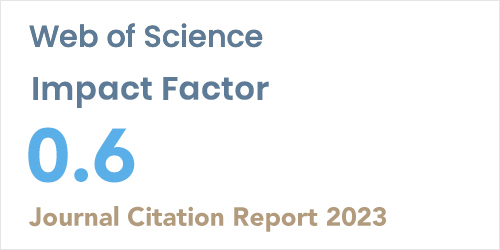ISSN: 1304-7191 | E-ISSN: 1304-7205
Volume: 41 Issue: 4 Year: 2023Estimating the Missing Value in One-Way Anova Under Long-Tailed Symmetric Error Distributions
1Sinop University, Department of Statistics, SINOP
2Ankara University, Department of Statistics, ANKARA
Sigma J Eng Nat Sci 2018; 36(2): 523-538

#### Abstract

In practice, missing values are widely seen and create serious problems in almost all statistical analysis. In this study, to deal with missing values, we propose estimators for missing value in one-way analysis of variance (ANOVA) when the distribution of error terms is long-tailed symmetric (LTS). We use methodologies known as maximum likelihood (ML), modified maximum likelihood (MML) and least squares (LS) in estimating missing value. Expectation and maximization (EM) algorithm is used for computing ML estimate of missing value. We compare the efficiencies of LS, ML and MML estimators of missing value via Monte Carlo simulation study. Simulation results show that ML estimator of missing value is the most efficient among the others. The usefulness of the proposed estimators is illustrated by peak discharge data example taken from civil engineering.